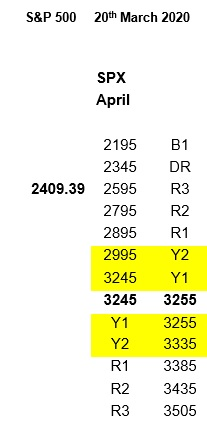# What Is Hedge Ratio?

The hedge ratio identifies the exact point for an index at which dynamic delta hedging will take place.

Dynamic hedging is defined as “delta hedging of a non-linear position with linear instruments like spot positions, futures or forwards. The deltas of the non-linear position and linear hedge position offset, yielding a zero delta overall. However, as the underlier’s value moves up or down, the delta of the non-linear position changes while that of the linear hedge does not. The deltas no longer offset, so the linear hedge has to be adjusted (increased or decreased) to restore the delta hedge. This continual adjusting of the linear position to maintain a delta hedge is called dynamic hedging”.

The Hedge Ratio simply does it for the entire market, or index.

So, in short, the Hedge Ratio identifies, on an index, in advance, where dynamic hedging will take place, and grades the degree of dynamic hedging (futures buying or selling)  to expect.

Starting with the NZ (Neutral Zone) where there is no Hedge Ratio, then up to YI and Y2, both minimal amounts and as such the most sensitive and therefore very fluid and so a good indication of whether the ratios are building or receding.

On the continuing exponential scale we then get the R ratio, the degrees of which are numbered in ascending magnitude 1, 2 and 3.

The next level is DR which is on its own as it has historically proved to be a significant enough level to turn markets around.

Above this is the B ratio level where markets can venture but in reality they shouldn’t.Here is an example of a Ratio Table.

At the top is the index it relates to, the “SPX”, better known as the S&P 500.

Then the date they have been calculated for, American style.

On the left, in bold, is the previous day’s close.

In the middle of the column, in bold, is 3245 to 3255, which represents this index’s zone.

This is where this index should be if deprived of every other influence except derivatives.

And, therefore, becomes very significant the closer the market comes to expiry.

Arranged top and bottom are the ratio levels.

Y1 and Y2 are highlighted in yellow to denote they are minimal, and therefore susceptible to change the quickest, meaning they can be early indicators of any potential directional bias.

On the right are the various levels of Hedge Ratio, but please bear in mind these are exponential.

Also, there is a rough guideline as to how a market may react when encountering them.

For the record the SPX all time high is 3393.52, the close that day was 3386.15, and this expiry ended on the 21st Feb, the Friday immediately prior to the start of the Caronavirus collapse on the Monday.Back on Friday the 20th March 2020 we published the Ratio Table opposite, which shows the levels for the forthcoming April expiry.

Therefore, the price on the left is the closing level of the S&P 500 on the 19th, as we publish before the market opens.

As we say above, beforehand we show where these exponential levels are, exactly, and how the market may react.

For the “B” ratios, the word we use is “dangerous”, as violent reactions can occur when hitting such a sudden huge amount of dynamic delta.

To date, the intraday low in the Caronavirus crash, in the SPX, stands at 2191.86.

For the record the Friday close was 2304.92, and the open on the 23rd was 2290.71 and the close 2237.40.

June 30th, 2017 by Richard